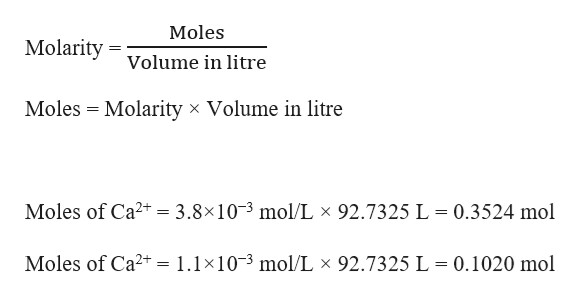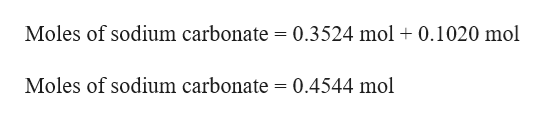# Sodium carbonate is often added to laundry detergents to soften hard water and make the detergent more effective. Suppose that a particular detergent mixture is designed to soften hard water that is 3.8×10−3M in Ca2+ and 1.1×10−3M in Mg2+ and that the average capacity of a washing machine is 24.5 gallons of water. 1gallon=3.785L If the detergent requires using 0.61 kg detergent per load of laundry, determine what percentage (by mass) of the detergent should be sodium carbonate in order to completely precipitate all of the calcium and magnesium ions in an average load of laundry water.Express your answer in percent to two significant figures.

Question
312 views
Sodium carbonate is often added to laundry detergents to soften hard water and make the detergent more effective. Suppose that a particular detergent mixture is designed to soften hard water that is 3.8×10−3M in Ca2+ and 1.1×10−3M in Mg2+ and that the average capacity of a washing machine is 24.5 gallons of water. 1gallon=3.785L

If the detergent requires using 0.61 kg detergent per load of laundry, determine what percentage (by mass) of the detergent should be sodium carbonate in order to completely precipitate all of the calcium and magnesium ions in an average load of laundry water.
check_circle

Step 1

Given,

Concentration of Ca2+ = 3.8×10−3 M = 3.8×10−3 mol/L

Concentration of Mg2+ = 1.1×10−3 M = 1.1×10−3 mol/L

1gallon = 3.785L

The average capacity of a washing machine = 24.5 gallons

= 24.5 × 3.785 L = 92.7325 L

Mass of detergent = 0.61 kg

= 0.61 × 1000 g = 610 g

Step 2

Moles of Ca2+ and Mg2+ can be calculated as:help_outlineImage TranscriptioncloseMoles Molarity Volume in litre Moles Molarity x Volume in litre Moles of Ca2 = 3.8x10-3 mol/L x 92.7325 L = 0.3524 mol Moles of Ca2 = 1.1x103 mol/L x 92.7325 L = 0.1020 mol fullscreen
Step 3

The reaction of sodium carbonate with calcium and magnesium ions to give precipitate can be written as:

Na2CO3 + Ca2+ → CaCO3 (s) + Na+

Na2CO3 + Mg2+ → MgCO3 (s) + Na+

1 mole of Ca2+ is required to precipitate 1 mole of Na2CO3

1 mole of Mg2+ is required to precipita...help_outlineImage TranscriptioncloseMoles of sodium carbonate = 0.3524 mol 0.1020 mol Moles of sodium carbonate = 0.4544 mol fullscreen

### Want to see the full answer?

See Solution

#### Want to see this answer and more?

Solutions are written by subject experts who are available 24/7. Questions are typically answered within 1 hour.*

See Solution
*Response times may vary by subject and question.
Tagged in

### Solutions